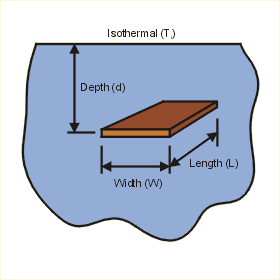Close

This page calculates the plate temperature for an isothermal (constant temperature) flat plate embedded in a semi-infinite medium with an applied isothermal temperature.The plate temperature Tp is calculated as:

Tp = Ti + q / (k x sf)

Where Ti is the isothermal temperature applied to the medium surrounding the plate, q is the heat flow, sf is the shape factor, and k is the conductivity of the medium.

The shape factor depends on the relationships between  the depth, width, and length of the plate. Three cases can be calculated.

Case 1:

When the depth of the plate is zero (the plate is at the surface), and the plate width is greater than the plate length, then the following equation is used:

sf = pi x W / ( ln (4 W / L) )

Case 2:

When the depth is much larger than the plate width (at least 10 times larger) and the plate width is greater than the plate length, then another equation can be applied:

sf = 2 pi x W / ( ln (4 W / L) )

Case 3:

When the plate width is much larger than the plate length (at least 10 times larger) and the depth of the embedded plate is at least twice the width, the shape factor is calculated as:

sf = 2 pi x W / ( ln (2 pi d / L)

Where W is the plate width, L is the plate length, and d is the plate depth.

References

Holman, J.P., Heat Transfer, 7th ed., McGraw Hill Book Company, New York, 1990. p 74 - 83